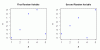I have forgotten

•http://facebook.com/
•https://www.google.com/accounts/o8/id
•https://me.yahoo.com

# Moments

#### Mean

Calculates the arithmetic mean of a given population
 template double mean (int n, T *data)

#### Avedev

Calculates the absolute deviation of a given set of data.
 template double avedev (int n, T *data)

#### Combin

Calculates the number of combinations for a given set of data.
 double combin (int n, int k)

#### Covariance

Calculates the covariance of a given set of data
 template double covariance (int n, T *data, T *data1)#### Squared Deviations

Calculates the sum of squared deviations of a given set of data.
 template double devsq (int n, T *data)

#### Fisher

Calculates Fisher transformation.
 double fisher (double x)

#### Fisher Inverse

Calculates the inverse of the Fisher transformation.
 double fisher_inv (double x)[inline]

#### Frequency

Calculates how often values occur within a given set of ranges.
 template std::vector frequency (int n, T *data, int m, T *bin)

#### Geometric

Calculates the geometric mean of a given population.
 template double geometric (int n, T *data)

#### Harmonic

Calculates the harmonic mean of a given population.
 template double harmonic (int n, T *data)

#### Kurtosis

Calculates the kurtosis of a given set of data.
 template double kurtosis (int n, T *data)

#### Mode

Finds the most common value in an array.
 template T mode (int n, T *data)

#### Pearson

Calculates the Pearson product for a given set of data.
 template double pearson (int N, T *data, T *data1)

#### Permut

Calculates the number of permutations for a given set of data.
 double permut (int n, int k)

#### Prob

Calculates the probability that values in a range are between two limits.
 template double prob (int n, T *data, double *prob, int x, int y=INT_MAX)

#### Rank

Calculates the rank of a number in a list of numbers.
 template int rank (int x, int n, T *data, bool ascending=true)

#### Skewness

Calculates the skewness of a given set of data.
 template double skewness (int n, T *data)

#### Smallest

Finds the smallest value of order k in the given array.
 template T smallest (int n, T *data, int k)

#### Trim Mean

Calculates the mean of the interior of data set.
 template double trimmean (int n, T *data, double p)

#### Variance

Calculates the variance of a given set of data.
 template double variance (int n, T *data, bool total = false)

#### Correlation

Calculates the correlation of a given set of data.
 templatedouble correlation (int n ,T *data ,T *data1)[inline]

#### Standard Deviation

Calculates the standard deviation of a given population.
 template double stdev (int n, T *data, bool total = false)

#### Largest

Finds the largest value of order k in the given array.
 templateT largest (int n, T *data, int k)[inline]

#### Median

Returns the median of the given population.
 template double median (int n, T *data)[inline]

#### Statistics

Calculates the statistics for a set of data that may change size frequently.
 template<...> class Statistics ()